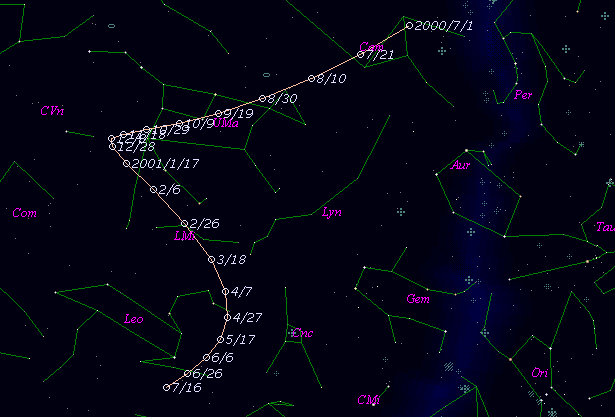# \$B%j%K%"WB@1(B

C/2001 A1 ( LINEAR )###\$B%W%m%U%#!<%k(B

 \$BH/8+F|(B 2001\$BG/(B1\$B7n(B7\$BF|(B \$BH/8+8wEY(B 17.7\$BEy(B \$BH/8+ Lincoln Laboratory Near-Earth Asteroid Research project

###\$B50F;MWAG(B

```   The following improved orbital elements by Kenji Muraoka, are
from 120 observations  2001 Jan. 7  to  Mar. 18, perturbations
by 9 Planets, Moon and 5 minor planets were taken into account.
The mean residual is +/- 0.76 arc seconds.

Epoch  =  2000 Sep. 13.0  TT       JDT = 2451800.5
T  =  2000 Sep. 17.59987       +/- 0.00685 (m.e.) TT
Peri. =  107.87879                +/- 0.00522
Node  =  339.59578                +/- 0.00113   (2000.0)
Incl. =   59.93876                +/- 0.00209
q  =    2.4062828              +/- 0.0001452 AU
e  =    0.9909158              +/- 0.0000835
1/a  =   +0.0037752              +/- 0.0000347 1/AU
( P  =   4311 years )
```

###\$B@1?^(B###\$B8wEYJQ2=(B

```        m1 = 11.4 + 5 log\$B&\$(B + 7.5 log r
```##### \$B50F;MWAG\$OB<2,7r<#;a\$N7W;;\$K\$h\$k\$b\$N\$G\$9!#(B \$B@1?^\$O(B StellaNavigator Ver.2.0 for Windows (\$B%"%9%H%m%"!<%D(B \$BJTCx(B / \$B%"%9%-!<=PHG6I4)(B) \$B\$G:n@.\$7\$?\$b\$N\$G\$9!#(B \$B8wEY%0%i%U\$O(BComet for Windows\$B\$G:n@.\$7\$?\$b\$N\$G\$9!#(B